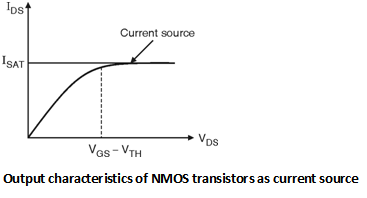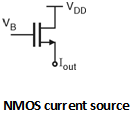Home > Analog CMOS Design > MOSFET Fundamentals > Current Source

Current Sources :

In order to use the MOSFET as a current source, we will have to operate it in saturation region.

According to the output characteristics of the MOSFET, the current in the saturation region is constant. (See Figure below). Therefore if we operate the MOSFET in saturation region it will work as a current source.

From Figure it can be seen that, if VDS is greater than the gate overdrive voltage i.e. (VGS â€“VTH) then the drain current flowing through the device is constant. This current is called as Saturation current denoted by ISAT.Figure below shows the current source circuit based on NMOS transistor. The condition to operate the transistor as a current source is that, the voltage VB should be applied with fixed baising voltage which will create the inversion channel of electrons.Once the inversion is created then the current will flow through the device and will act as a current source when VDD is greater than the gate overdrive voltage. i.e. (VB â€“ VTH). The important application of current source is it can work as a load resistor in amplifier circuits with larger load resistance value.